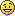# Automatic axis range for 2D and 3D histograms

Hi,

I am trying to fill a 2D histogram which have unknown range of X-value, but the range of Y-axis is already known. I use the automatic axis range feature, namely set both the upper and lower limit of X-axis to 0, like this

``TH2* h2 = new TH2D("h2", "2D hist", 100, 0, 0, 100, 0, 1); ``

it works fine for the X-axis. However, it also set automatic limits for Y-axis! Having a check of the source of TH2::BufferEmpty(), I found that it does not distinguish which axis should be automatic, and just reset both. I think it could be smarter to have separated treatment for X and Y axis, like this: (part of BufferEmpty(), not yet tested)

``````//...
/// finished checkings

#warning just a demo, not yet tested!
if (CanExtendAllAxes() || fXaxis.GetXmax() <= fXaxis.GetXmin()) {
//find min, max of entries in buffer
Double_t xmin = fBuffer;
Double_t xmax = xmin;
for (Int_t i=1;i<nbentries;i++) {
Double_t x = fBuffer[3*i+2];
if (x < xmin) xmin = x;
if (x > xmax) xmax = x;
}
if (fXaxis.GetXmax() <= fXaxis.GetXmin()) {
THLimitsFinder::GetLimitsFinder()->FindGoodLimits(this,xmin,xmax);
} else {
fBuffer = 0;
Int_t keep = fBufferSize; fBufferSize = 0;
if (xmin <  fXaxis.GetXmin()) ExtendAxis(xmin,&fXaxis);
if (xmax >= fXaxis.GetXmax()) ExtendAxis(xmax,&fXaxis);
fBuffer = buffer;
fBufferSize = keep;
}
}

if (CanExtendAllAxes() || fYaxis.GetXmax() <= fYaxis.GetXmin()) {
//find min, max of entries in buffer
Double_t ymin = fBuffer;
Double_t ymax = ymin;
for (Int_t i=1;i<nbentries;i++) {
Double_t y = fBuffer[3*i+3];
if (y < ymin) ymin = y;
if (y > ymax) ymax = y;
}
if (fYaxis.GetXmax() <= fYaxis.GetXmin()) {
/// THLimitsFinder::GetLimitsFinder()->FindGoodLimits(this,xmin,xmax,ymin,ymax);
/// fork FindGoodLimits()
/// or we can modify FindGoodLimits()
TAxis *yaxis = fYaxis;
Int_t binsx = fXaxis->GetNbins(),newbinsy;
Double_t xmin = fXaxis->GetXmin(), xmax = fXaxis->Xmax();

if (ymin >= ymax) {
if (yaxis->GetLabels()) {ymin  = 0; ymax  = ymin +yaxis->GetNbins();}
else                    {ymin -= 1; ymax += 1;}
}

THLimitsFinder::OptimizeLimits(yaxis->GetNbins(),
newbinsy,ymin,ymax,
yaxis->TestBit(TAxis::kIsInteger));

h->SetBins(binsx,xmin,xmax, newbinsy,ymin,ymax);
/// end of insertion
} else {
fBuffer = 0;
Int_t keep = fBufferSize; fBufferSize = 0;
if (ymin <  fYaxis.GetXmin()) ExtendAxis(ymin,&fYaxis);
if (ymax >= fYaxis.GetXmax()) ExtendAxis(ymax,&fYaxis);
fBuffer = buffer;
fBufferSize = keep;
}
}

/// then fill the buffer to histogram and empty the buffer
//...``````

The same problem exists in TH3::BufferEmpty().

Hi. We’re checking into your question. Will update here later.

Hi,

Your requests make sense. since the automatic binning works for all histogram axis. I will add this as a new feature request for next release

Best Regards

Lorenzo